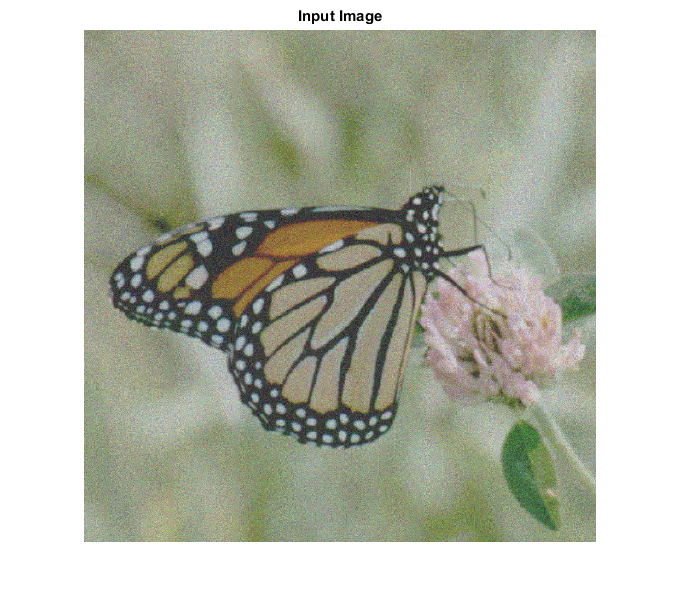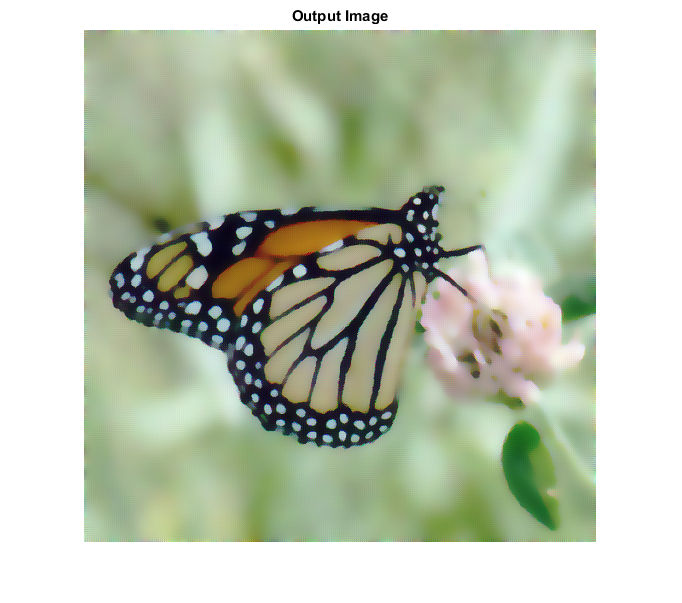ad_filter_cvip() - anisotropic diffusion filter.

## SYNTAX

`out = ad_filter_cvip( I,iter,lambda,K, opt)`

Input Parameters Include :

• I - Input image can be gray image or rgb image of MxN size.
• iter - Number of iterations.
• lambda - Smoothing per iteration.
• K - Edge threshold.
• opt - Coefficient functions suggested by Perona & Malik. 1 - c(x,y,t) = exp(-(|nablaI|/K).^2), 2 - c(x,y,t) = 1./(1 + (|nablaI|/K).^2),

Output Parameters Include :

• out - The output image after filtering. An image with the same size as the input image.

## DESCRIPTION

Anisotropic diffusion filter runs an iterative algorithm on the input image which tries to diffuses the gray levels in similar areas without removing the borders. The argument opt determines which coefficient function is used in this mehtod.The coefficient functions suggested by Perona & Malik are:

`        1 - c(x,y,t) = exp(-(||nablaI||/K).^2),`
`        2 - c(x,y,t) = 1./(1 + (||nablaI||/K).^2),`

You can control smoothing per iteration with lambda and edge threshold with K.

This is an edge preserving,smoothing filter.The filter is anisotropic which means it will respond differently in different directions,based on image characteristics.This will enable the filter to stop the diffusion process at the edges,while still smoothing in areas of homogeneity.It operates by a diffusion process that iteratively smoothes the image.At each iteration of the filter more smoothing is performed and more image detail is lost.ad_filter provides a more natural looking image.

## REFERENCE

1.Scott E Umbaugh. DIGITAL IMAGE PROCESSING AND ANALYSIS: Applications with MATLAB and CVIPtools, 3rd Edition.

2.P.Perona and J.Malik. Scale-Space and Edge Detection Using Anisotropic Diffusion. IEEE Transactions on Pattern Analysis and Machine Intelligence, 12(7):629-639, July 1990.

## EXAMPLE

```% Read Image

% Number of iterations

iter = 20;

% Smoothing per iteration

lambda = 1;

% Edge threshold

K = 6;

% coefficient functions

opt = 2;

% Call function

out = ad_filter_cvip( I,iter,lambda,K, opt);

% Display Input Image

figure;imshow(I);title('Input Image');

% Display Output Image

figure; imshow(remap_cvip(out));title('Output Image');
```## CREDITS

Author: Mehrdad Alvandipour, March 2017